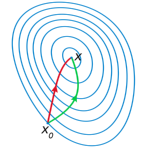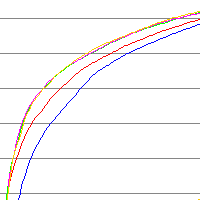# Logistic Regression and Optimization BasicsLogistic regression uses a sigmoid (logistic) function to pose binary classification as a curve fitting (regression) problem. It can be a useful technique, but more importantly it provides a good example to illustrate the basics of nonlinear optimization. I’ll show how to solve this problem iteratively with both gradient descent and Newton’s method as well as go over the Wolfe conditions that we’ll satisfy to guarantee fast convergence. These are the prerequisites for the upcoming neural network articles!
Continue reading Logistic Regression and Optimization Basics

# Classification ValidationIt’s important to know how well your models are likely to work in the real world. In this article I’ll be discussing basic testing procedures  for classification problems such as generating receiver operating characteristics and confusion matrices as well as performing cross validation. We’ll also take a look at the effect of over-fitting and under-fitting models.
Continue reading Classification Validation